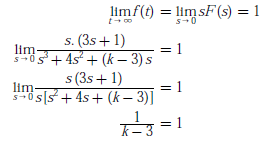# Engineering Mathematics - Online Test

Q1. The order of the differential equationAnswer : Option B
Explaination / Solution:

The highest derivative terms present in DE is of 2nd order.

Q2. The eigen values of a skew-symmetric matrix are
Answer : Option C
Explaination / Solution:

Eigen value of a Skew-symmetric matrix are either zero or pure imaginary in conjugate pairs.

Q3. The trigonometric Fourier series for the waveform f (t) shown below contains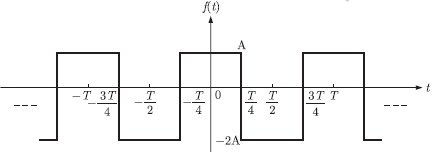Answer : Option C
Explaination / Solution:

For a function x(t) trigonometric fourier series isFor an even function x(t),Bn = 0
Since given function is even function so coefficient Bn = 0, only cosine and constant
terms are present in its fourier series representation.
Constant term :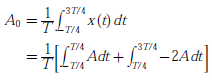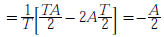Constant term is negative.

Q4. A function n(x) satisfied the differential equation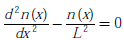where L is a constant. The boundary conditions are :n(0) = K and n() = 0. The solution to this equation is
Answer : Option D
Explaination / Solution:

Given differential equation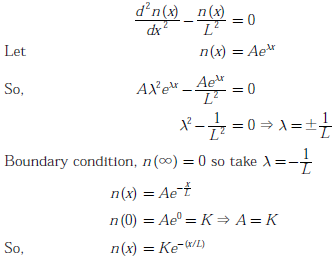Q5. Consider the z -transform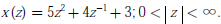The inverse z -transform x[n] is
Answer : Option A
Explaination / Solution: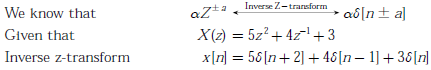Q6. If ey = x1/x , then y has a
Answer : Option A
Explaination / Solution: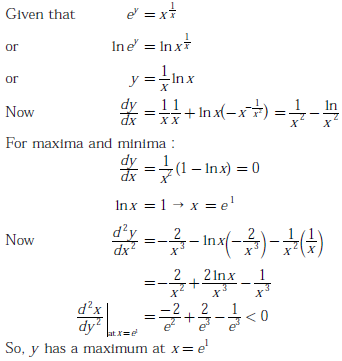Q7. A fair coin is tossed independently four times. The probability of the event “the number of time heads shown up is more than the number of times tail shown up”
Answer : Option D
Explaination / Solution:
No Explaination.

Q8. If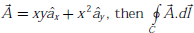over the path shown in the figure is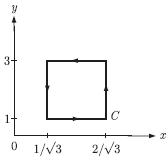Answer : Option C
Explaination / Solution: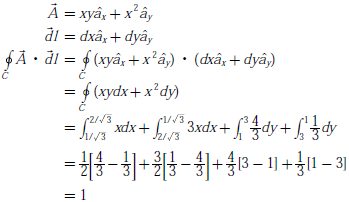Q9.
Consider differential equation with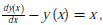the initial condition y(0) = 0. Using Euler’s first order method with a step size of 0.1, the value of y(0.3) is
Answer : Option B
Explaination / Solution:

Taking step size h = 0.1, y(0) = 0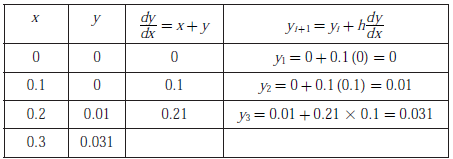From table, at x = 0.3,y(x = 0.3) = 0.031

Q10. Given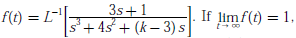then the value of k is
Answer : Option D
Explaination / Solution: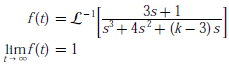By final value theorem